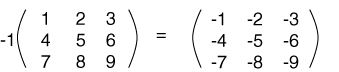Each element in the result is the negative of the original, as seen below.

Negative of a Matrix

The negation of a matrix is formed by negating each element of the matrix:

-A = -1A

So, for example:It will not surprise you that:

A + (-A)  = 0

(Notice that that last zero is a bold-faced zero, designating the zero matrix.)

QUESTION 12:

Look at the above fact. Can you think of a way to define matrix subtraction?